﻿ 船用大载荷升降设备控制系统设计与仿真
 舰船科学技术2017, Vol. 39Issue (12): 167-170PDF

1. 中国船舶重工集团公司第七一三研究所，河南 郑州 450015;
2. 海军驻郑州地区军事代表室，河南 郑州 450015

Design and simulation for large load ship lifter control system
LI Yuan-zhe1, WANG Kai2
1. The 713 Research Institute of CSIC, Zhengzhou 450015, China;
2. Naval Representative Office in Zhengzhou Region, Zhengzhou 450015, China
Abstract: This article proposed a servo control model for the flexibility feature of steel wire rope. According to the system simulation and the improvement of position loop PID controller, the position error can be eliminated , and the high-precision controlling demand is able to be satisfied.
Key words: large load     dual-motor     servo control     PID control
0 引　言

1 双电机升降装置伺服控制系统的结构组成

2个电机采用较常用的主从控制结构，即主电机工作于位置环，从电机工作于电流环。其控制系统结构详见图1，主电机和从电机具备相互独立的电流环，并设计一个电流调节器使其稳定且无静差。主电机设计在电流环外部设计一个速度环调节器，使速度环稳定且无静差，其输出电流给定同时发送给主从电机。主电机同样设计了一个位置调节器，对位置误差进行计算。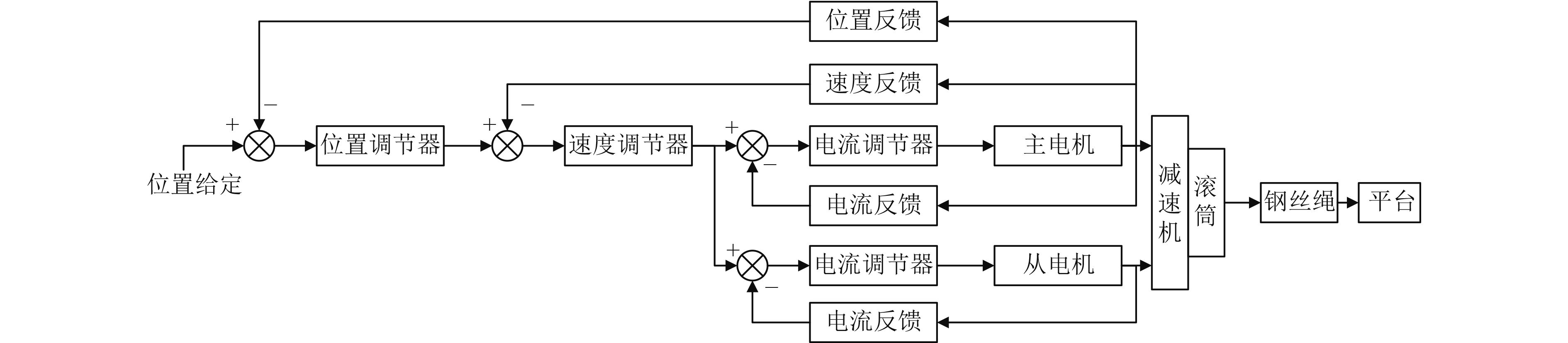图 1 双电机伺服驱动控制系统组成 Fig. 1 The components of dual-motor servo control system
2 双电机伺服驱动系统的数学模型

 $U = RI + L\frac{{{\rm d}I}}{{{\rm d}t}} + V \text{，}$ (1)

 ${T_M} = {K_M}I \text{，}$ (2)

 $V = {K_\varOmega }{\varOmega _M} \text{，}$ (3)

 ${T_M} = ({J_M} + {J_L})\frac{{{{\rm d}^2}{\theta _M}}}{{{\rm d}{t^2}}} + ({D_M} + {D_L})\frac{{{\rm d}{\theta _M}}}{{{\rm d}{t^2}}} + {T_L} \text{，}$ (4)

 $\frac{{U\left( {{s}} \right) - V\left( {{s}} \right)}}{{{{R}} + {{Ls}}}} = {{I}}\left( {{S}} \right) \text{，}$ (5)
 $V\left( {{s}} \right) = {{{K}}_{{M}}}{\varOmega _{{M}}}\left( {{s}} \right) \text{，}$ (6)
 ${{{T}}_{{M}}}\left( {{s}} \right) = {{{K}}_{{M}}}\left( {{s}} \right){{I}}\left( {{s}} \right) \text{，}$ (7)
 $\frac{{{{{T}}_{{M}}}\left( {{s}} \right) - {{{T}}_{{L}}} - ({D_L} + {D_M}){\varOmega _M}\left( s \right)}}{{({J_M} + {J_L})s}} = {\varOmega _M}\left( s \right) \text{。}$ (8)

 ${{{G}}_{{L}}}\theta {{R}} = {{kx}} \text{。}$ (9)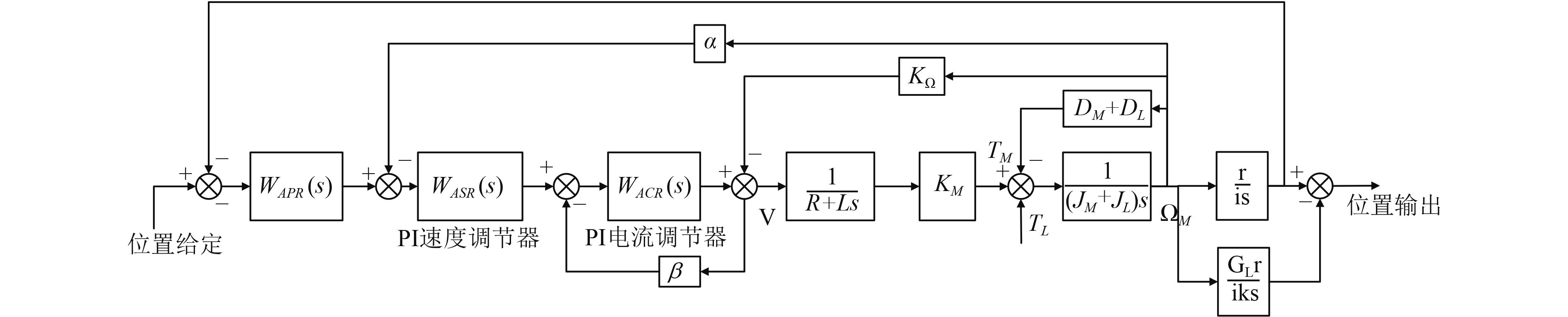图 2 控制系统原理框图 Fig. 2 The functional block diagram of control system
3 改进的控制系统模型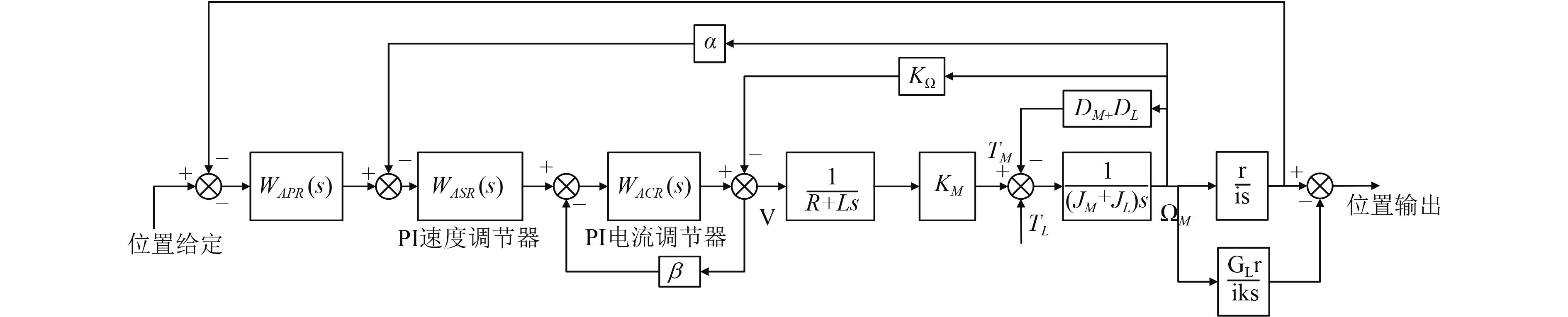图 3 改进的控制系统原理框图 Fig. 3 The modified functional block diagram of control system
4 控制系统仿真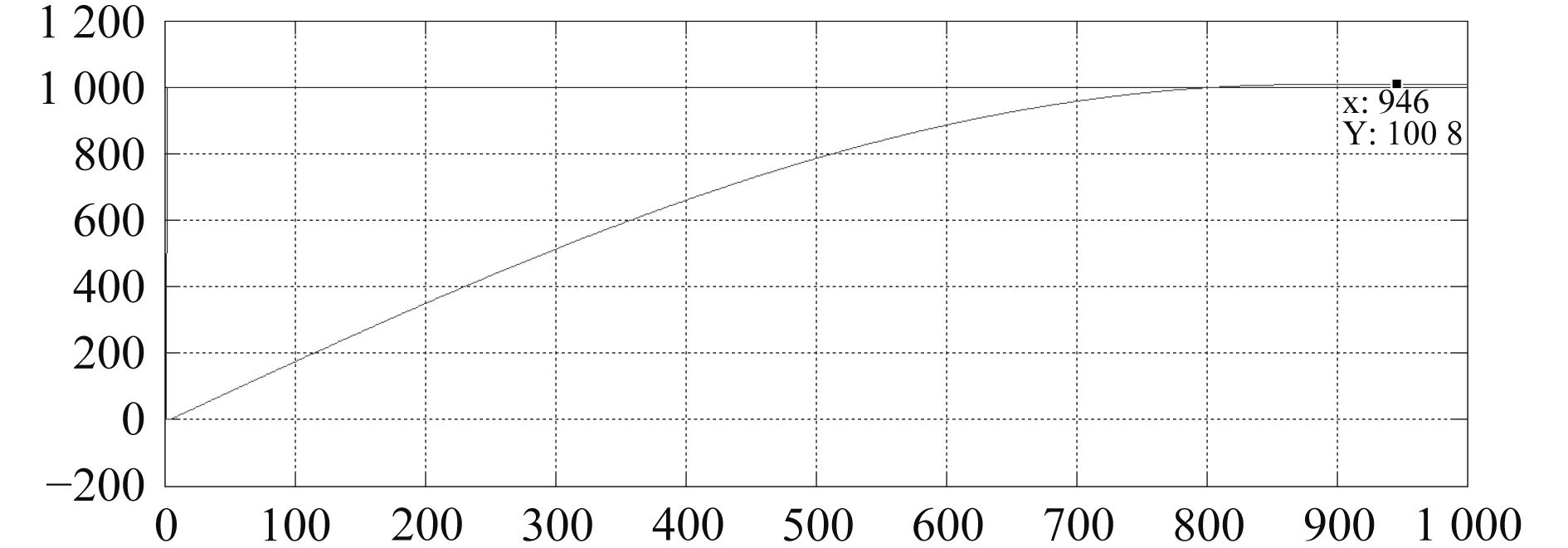图 4 额定载荷下的位置阶跃响应曲线-有静差 Fig. 4 The position step response curve on rated load-steady-with state error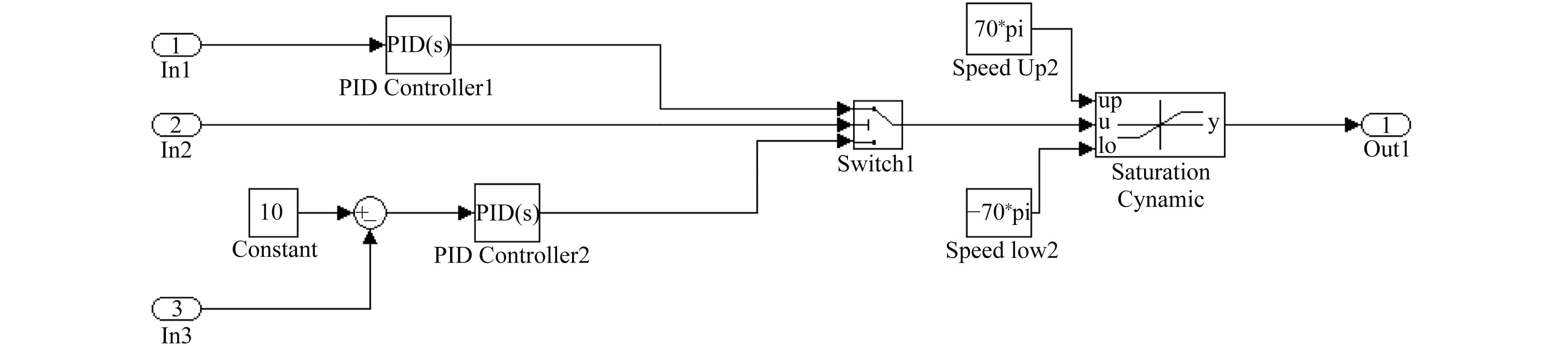图 5 改进的位置环PID控制器结构仿真图 Fig. 5 The simulation diagram of modified position-loop PID controller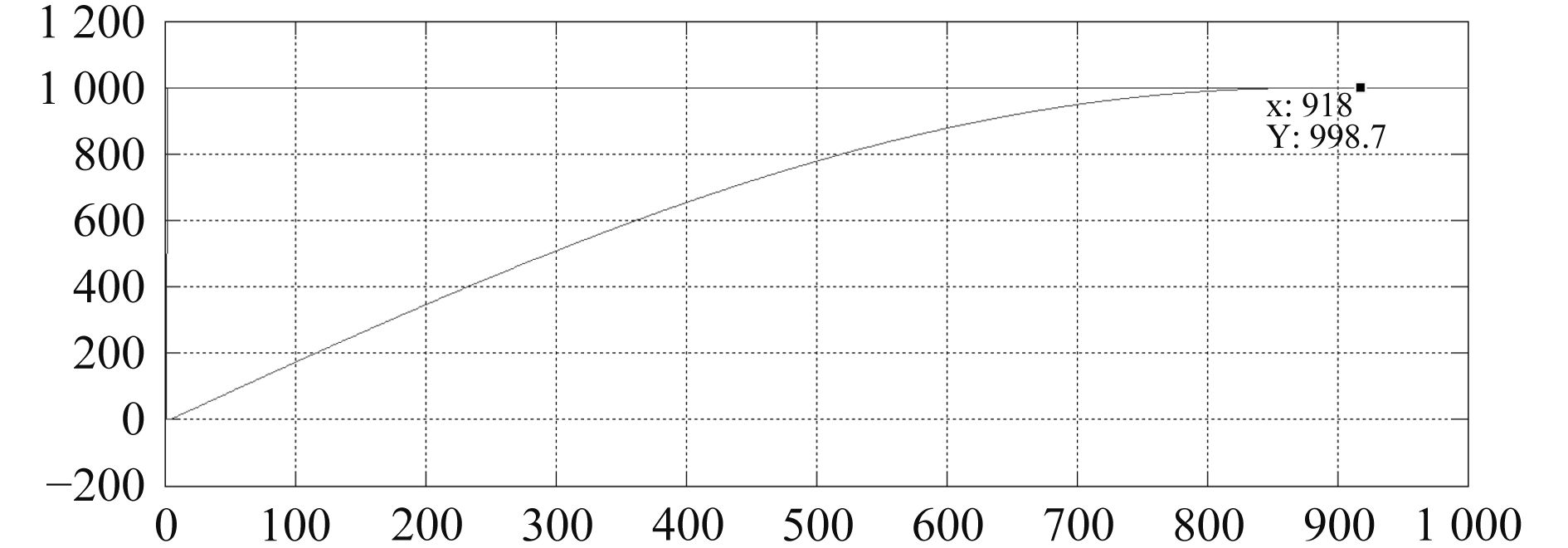图 6 额定载荷下的位置阶跃响应曲线-无静差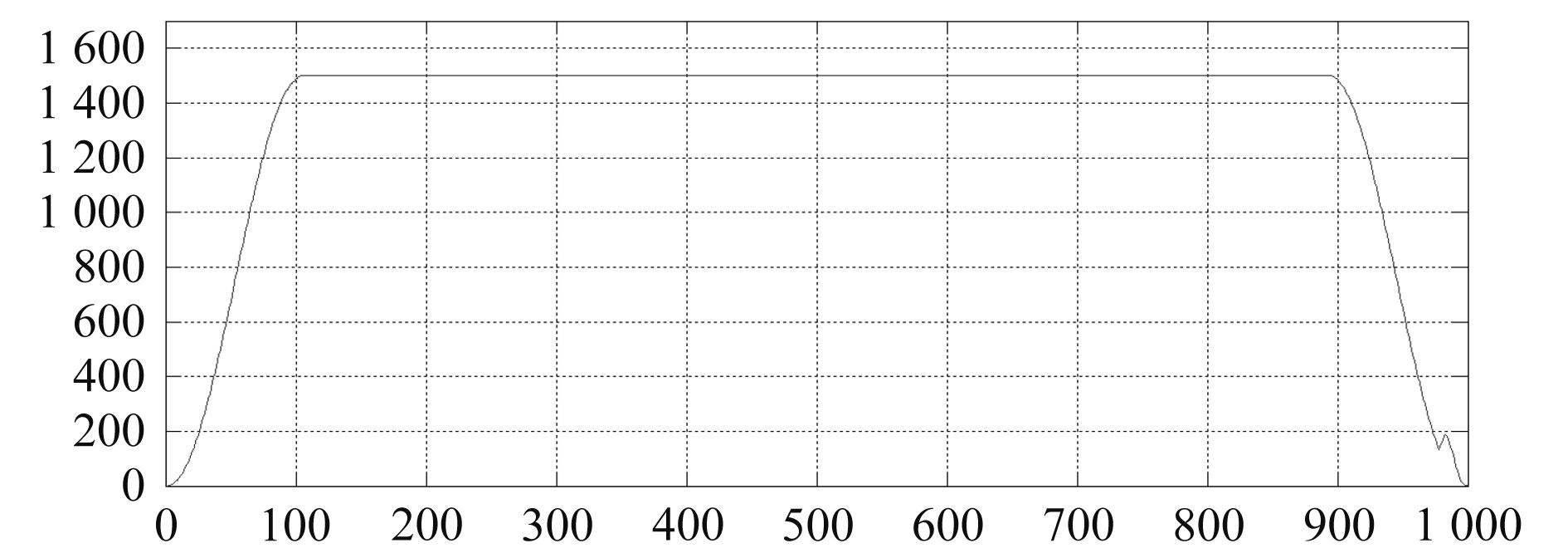图 7 电机运行速度曲线 Fig. 7 The speed curve of motor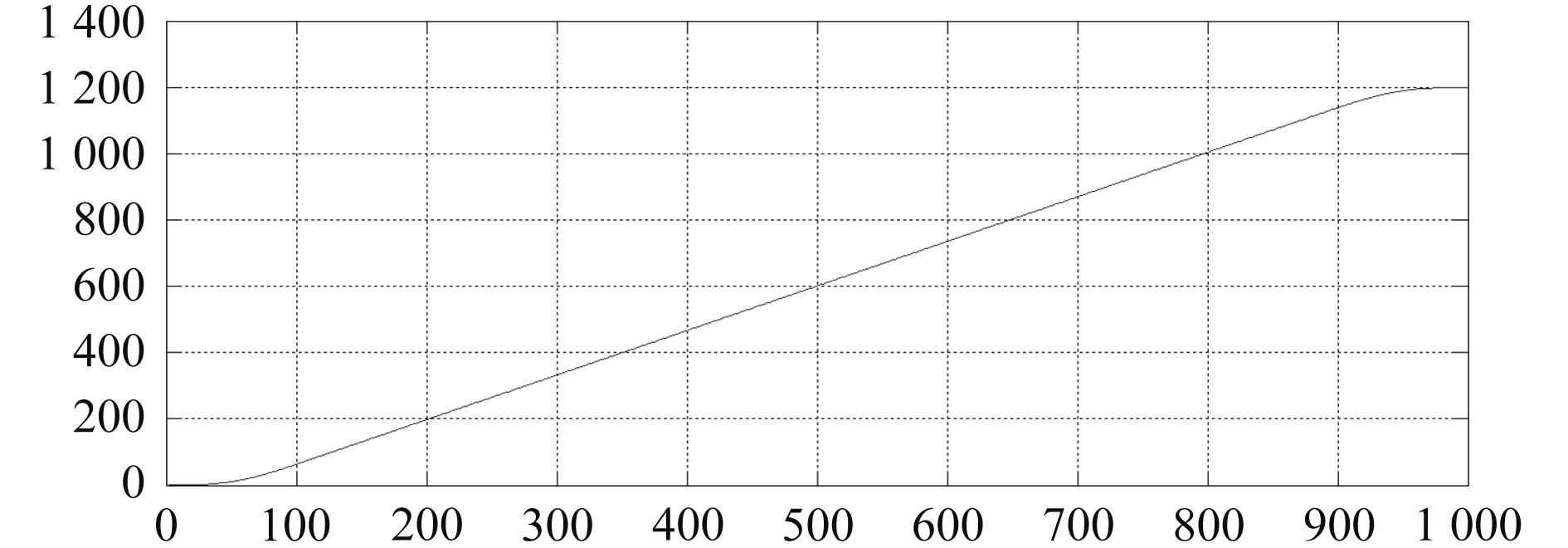图 8 平台位置曲线 Fig. 8 The position curve of platform
5 结　语

  陈伯时. 电力拖动自动控制系统[M]. 北京: 机械工业出版社, 1999: 25.  薛定基, 陈阳泉. 基于Matlab/Simulink的系统仿真技术应用[M]. 北京: 清华大学出版社, 2002.  赵海波. 双电机同步联动伺服系统的设计与分析[D]. 南京: 南京理工大学, 2006.  胡寿松. 自动控制原理[M]. 第四版. 北京: 科学出版社, 2001.